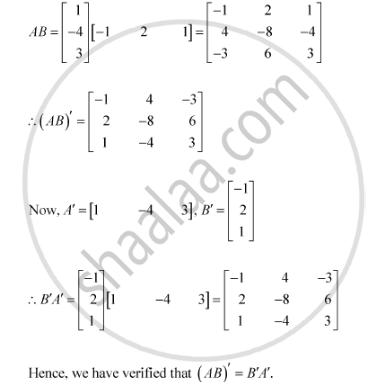Share

# For the Matrices A And B, Verify that (Ab)′ = B'A' Where a =Matric [(1),(-4), (3)], B = Matric [-1, 2 1] - CBSE (Science) Class 12 - Mathematics

ConceptSymmetric and Skew Symmetric Matrices

#### Question

For the matrices A and B, verify that (AB)′ = B'A' where

A =[(1),(-4), (3)], B = [-1, 2 1]

#### SolutionIs there an error in this question or solution?

#### Video TutorialsVIEW ALL 

Solution For the Matrices A And B, Verify that (Ab)′ = B'A' Where a =Matric [(1),(-4), (3)], B = Matric [-1, 2 1] Concept: Symmetric and Skew Symmetric Matrices.
S